# Texas Go Math Grade 1 Lesson 1.7 Answer Key Model, Read, and Write Numbers from 100 to 120

Refer to our Texas Go Math Grade 1 Answer Key Pdf to score good marks in the exams. Test yourself by practicing the problems from Texas Go Math Grade 1 Lesson 1.7 Answer Key Model, Read, and Write Numbers from 100 to 120.

## Texas Go Math Grade 1 Lesson 1.7 Answer Key Model, Read, and Write Numbers from 100 to 120

Essential Question
How can you model, read, and write numbers from 100 to 120?
Explanation:
10 tens = 100
12 tens = 120

Explore
How many seashells are there?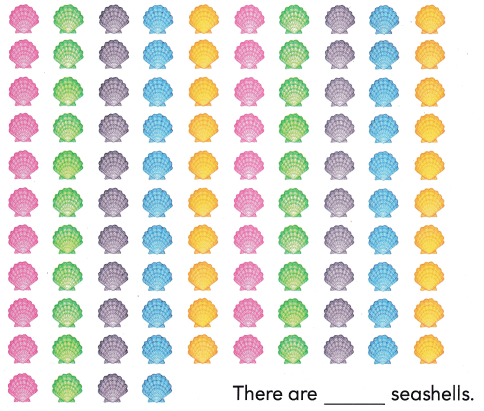Explanation: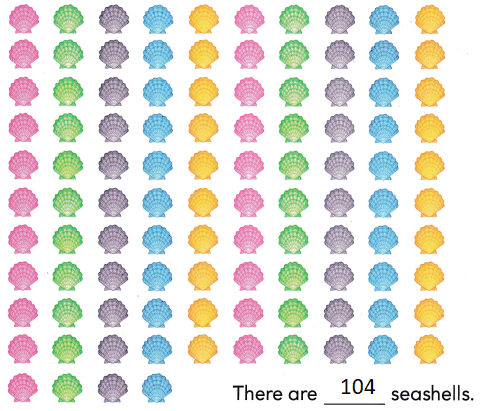10 tens + 4 ones = 104

For The Teacher

• Read the problem. Heidi has this collection of seashells. How many seashells does Heidi have?
Explanation:
10 tens + 4 ones = 104
104 seashells that Heidi have

Math Talk
Mathematical processes
How did you decide how many seashells there are? Explain.
Explanation:
10 tens + 4 ones = 104
By counting the columns
we decide that there are 10 columns
10 tens = 100

Model and Draw

1 hundred 0 tens 1 one is 101,Explanation:
The sum of hundreds , tens and ones
100 + 0 + 1 = 101

1 hundred 1 ten 1 one is III.Explanation:
The sum of hundreds , tens and ones

Share and Show

Useto model the number. Write the numbers.

Question 1.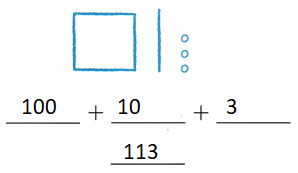Explanation:
The sum of hundreds , tens and ones
100 +10 + 3 = 113

Question 2.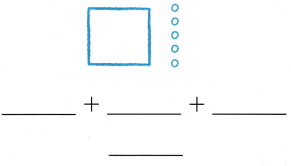Explanation:
The sum of hundreds , tens and ones
100 + 0 + 5 = 105

Question 3.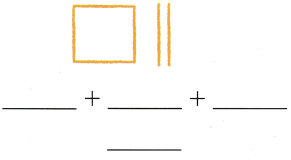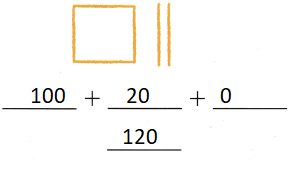Explanation:
The sum of hundreds , tens and ones
100 + 20 + 0 = 120

Question 4.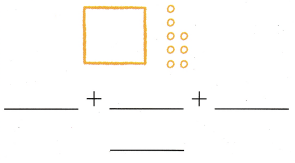Explanation:
The sum of hundreds , tens and ones
100 + 0 + 8 = 108

Problem Solving

Use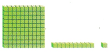to model the number. Write the numbers.

Question 5.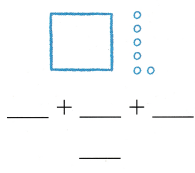Explanation:
The sum of hundreds , tens and ones
100 + 6 = 106

Question 6.Explanation:
The sum of hundreds , tens and ones
100 + 10 + 5 = 115

Question 7.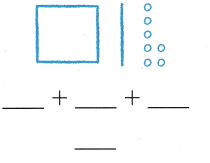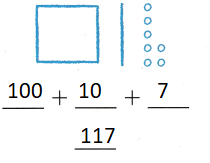Explanation:
The sum of hundreds , tens and ones
100 + 10 + 7 = 117

Choose a way to solve.

Draw or write to explain.

Question 8.
H.O.T. Lee can make 11 groups of 10 marbles and has 2 marbles left over. How many marbles does Lee have?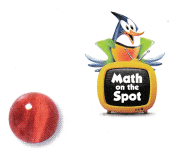____ marbles
112 marbles
Explanation:
11 groups of 10 marbles = 110
2 left over = 112

Question 9.
H.O.T. Multi-Step Nate can make 1 group of 100 pebbles. Lucy can make 1 group of 10 pebbles. Dylan has 6 pebbles. How many pebbles do they have?
____ pebbles
116 pebbles
Explanation:
Nate can make 1 group of 100 pebbles. Lucy can make 1 group of 10 pebbles. Dylan has 6 pebbles.
100 + 10 + 6 = 116

Question 10.
Use Tools Joe collects pennies. He can make 11 groups of 10 pennies. How many pennies does Joe have?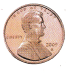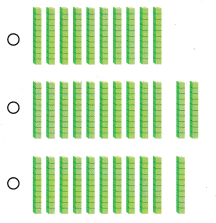Explanation:
Bubbled the correct option
11 tens

Question 11.
Cindy collects buttons. She can make 3 groups of 10 buttons and one more group of 4 buttons. How many buttons does Cindy have?(A) 14
(B) 34
(C) 44
Explanation:
make 3 groups of 10 buttons = 30
one more group of 4 buttons. = 4
30 + 4 = 34

Question 12.
Texas Test Prep Useto model the number. What number does the model show?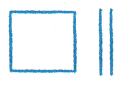(A) 102
(B) 120
(C) 110
Explanation:
100 +20 + 0 = 120
1 hundred 2 tens and 0 ones

### Texas Go Math Grade 1 Lesson 1.7 Homework and Practice Answer Key

Useto model the number. Write the numbers.

Question 1.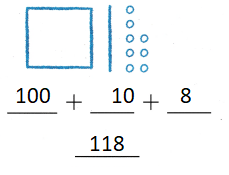Explanation:
The sum of hundreds , tens and ones
100 + 10 + 8 = 118

Question 2.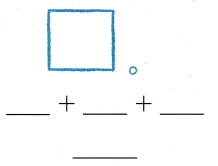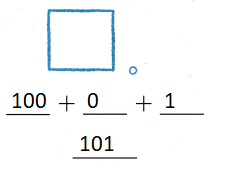Explanation:
The sum of hundreds , tens and ones
100 + 0 + 1 = 101

Question 3.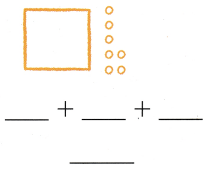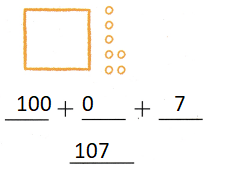Explanation:
The sum of hundreds , tens and ones
100 + 0 + 7 = 107

Question 4.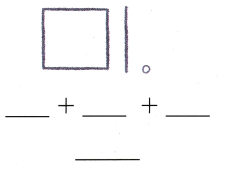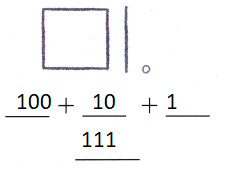Explanation:
The sum of hundreds , tens and ones
100 +10 + 1 = 111

Problem Solving

Choose a way to solve. Draw or write to explain.

Question 5.
Multi-Step Janelle has 10 groups of 10 crayons and 3 crayons left over. How many crayons does Janelle have?
__ crayons
103 crayons
Explanation:
10 groups of 10 crayons = 100
3 crayons left over = 3
100 + 3 = 103

Texas Test Prep

Lesson Check

Question 6.
A store clerk puts carrots into bunches. Each bunch contains 10 carrots. The clerk makes II bunches. Which model shows the number of carrots?Explanation:
Bubbled the correct option.
11 bunches = 100 + 10 = 110

Question 7.
A farmer picked carrots. He drew a quick picture to show the number of carrots he picked. What number does the picture show?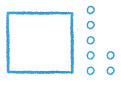(A) 107
(B) 117
(C) 127
Explanation:
100 + 7 = 107
107 is the number that the picture show

Question 8.
Which number does the model show?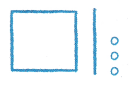(A) 103
(B) 113
(C) 131